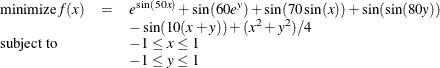An Optimization Problem with Many Local Minima

Consider the following optimization problem:The objective function is highly nonlinear and contains many local minima. The NLP solver provides you with the option of searching the feasible region and identifying local minima of better quality. This is achieved by writing the following SAS program:

proc optmodel;
var x >= -1 <= 1;
var y >= -1 <= 1;
min f = exp(sin(50*x)) + sin(60*exp(y)) + sin(70*sin(x)) + sin(sin(80*y))
- sin(10*(x+y)) + (x^2+y^2)/4;
solve with nlp / multistart seed=94245 msmaxstarts=30;
quit;


The MULTISTART option is specified, which directs the algorithm to start the local solver from many different starting points. The SAS log is shown in Figure 8.5.

Figure 8.5: Progress of the Algorithm as Shown in the Log

 NOTE: Problem generation will use 4 threads. NOTE: The problem has 2 variables (0 free, 0 fixed). NOTE: The problem has 0 linear constraints (0 LE, 0 EQ, 0 GE, 0 range). NOTE: The problem has 0 nonlinear constraints (0 LE, 0 EQ, 0 GE, 0 range). NOTE: The OPTMODEL presolver removed 0 variables, 0 linear constraints, and 0 nonlinear constraints. NOTE: Using analytic derivatives for objective. NOTE: The NLP solver is called. NOTE: The Interior Point algorithm is used. NOTE: The MULTISTART option is enabled. NOTE: The deterministic parallel mode is enabled. NOTE: The Multistart algorithm is executing in single-machine mode. NOTE: The Multistart algorithm is using up to 4 threads. NOTE: Random number seed 94245 is used. Best       Local  Optimality    Infeasi-  Local  Local Start    Objective   Objective       Error      bility  Iters  Status 1   -1.8567821  -1.8567821        5E-7           0      3  Optimal 2   -2.9390086  -2.9390086        5E-7           0      3  Optimal 3   -2.9390086  -2.6561783        5E-7           0      3  Optimal 4   -3.3068686  -3.3068686        5E-7           0      3  Optimal 5   -3.3068686  -2.2355863        5E-7           0      3  Optimal 6   -3.3068686  -1.9014527  6.14439E-7  6.14439E-7      4  Optimal 7   -3.3068686  -0.4808983        5E-7           0      3  Optimal 8   -3.3068686  -1.5173191        5E-7           0      4  Optimal 9   -3.3068686   -2.076195        5E-7           0      4  Optimal 10   -3.3068686  -2.1930943        5E-7           0      5  Optimal 11   -3.3068686  -0.7857749        5E-7           0      5  Optimal 12   -3.3068686  -0.4033346        5E-7           0      3  Optimal 13   -3.3068686  -0.5926643        5E-7           0      4  Optimal 14   -3.3068686  -2.0402058        5E-7           0      4  Optimal 15   -3.3068686  -2.9525781        5E-7           0      4  Optimal 16 * -3.3068686  -1.3289057        5E-7           0      4  Optimal 17   -3.3068686  -1.5650191        5E-7           0      7  Optimal 18   -3.3068686   -1.404132        5E-7           0      3  Optimal 19   -3.3068686  -2.4632393        5E-7           0      3  Optimal 20   -3.3068686  -2.4541355        5E-7           0      4  Optimal 21   -3.3068686  -2.5171432        5E-7           0      5  Optimal 22   -3.3068686  -1.3559281  8.74345E-7  8.74345E-7      3  Optimal 23   -3.3068686   -1.031811        5E-7           0      4  Optimal 24   -3.3068686  -1.0823455        5E-7           0      5  Optimal 25   -3.3068686   -2.387082        5E-7           0      4  Optimal 26   -3.3068686  -0.6723829        5E-7           0      4  Optimal 27   -3.3068686  -1.2265443        5E-7           0      4  Optimal 28   -3.3068686  -0.6391886        5E-7           0      4  Optimal 29   -3.3068686  -0.9393803        5E-7           0      4  Optimal 30   -3.3068686  -0.5519164        5E-7           0      3  Optimal NOTE: The Multistart algorithm generated 320 sample points. NOTE: 30 distinct local optima were found. NOTE: The best objective value found by local solver = -3.306868647. NOTE: The solution found by local solver with objective = -3.306868647 was returned.

The SAS log presents additional information when the MULTISTART option is enabled. The first column counts the number of restarts of the local solver. The second column records the best local optimum that has been found so far, and the third through sixth columns record the local optimum to which the solver has converged. The final column records the status of the local solver at every iteration.

The SAS output is shown in Figure 8.6.

Figure 8.6: Problem Summary and Solution Summary

The OPTMODEL Procedure

Problem Summary
Objective Sense Minimization
Objective Function f
Objective Type Nonlinear

Number of Variables 2
Bounded Above 0
Bounded Below 0
Bounded Below and Above 2
Free 0
Fixed 0

Number of Constraints 0

Performance Information
Execution Mode Single-Machine

Solution Summary
Solver Multistart NLP
Algorithm Interior Point
Objective Function f
Solution Status Optimal
Objective Value -3.306868647

Number of Starts 30
Number of Sample Points 320
Number of Distinct Optima 30
Random Seed Used 94245
Optimality Error 5E-7
Infeasibility 0

Presolve Time 0.00
Solution Time 3.57